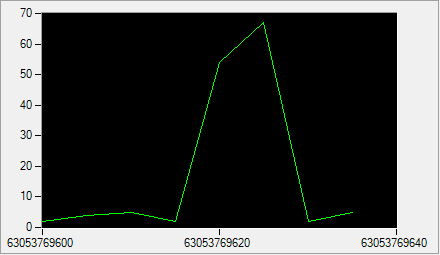From 9:00 AM - 12:00 PM CDT on Saturday, June 6th, ni.com will be undergoing system upgrades that may result in temporary service interruption.

We appreciate your patience as we improve our online experience.

# How Do I Display Timing Information When Plotting Waveforms?

Measurement Studio 2012 for Visual Studio 2010 Help

Edition Date: August 2012

Part Number: 372636F-01

»View Product InfoDownload Help (Windows Only)

## What Is The Default Behavior for Plotting Waveforms?

The PlotWaveform method uses DefaultWaveformPlotOptions to configure plotting. The default value of AnalogWaveformPlotDisplayMode is set to Samples.

When AnalogWaveformPlotDisplayMode is set to Samples and the Range on the x axis is set to 0, the waveform graph ignores timing information during plotting. If you set the x axis labels to the DateTime or the ElapsedTime format, time on the graph starts at 01/01/0001 12:00:00 AM.Note: This topic explains plotting and timing information for the waveform graph. Plotting complex waveforms is similar.

## How Do I Display Timing Information When Plotting Waveforms?

You display timing information when plotting a waveform with the following steps:

## How Can I Enable Timing Information on the Graph?

You enable timing information on the graph in one of two ways:

### Option 1

1. Create an AnalogWaveformPlotOptions object with Time.
2. Set DefaultWaveformPlotOptions to the AnalogWaveformPlotOptions object.
VB.NET
```waveformPlot1.DefaultWaveformPlotOptions = New AnalogWaveformPlotOptions(AnalogWaveformPlotDisplayMode.Time, AnalogWaveformPlotScaleMode.Raw)

waveformGraph1.PlotWaveform(Of Double)(myWaveform)```
C#
```waveformPlot1.DefaultWaveformPlotOptions = new AnalogWaveformPlotOptions(AnalogWaveformPlotDisplayMode.Time, AnalogWaveformPlotScaleMode.Raw);

waveformGraph1.PlotWaveform<double>(myWaveform);```

### Option 2

1. Create an AnalogWaveformPlotOptions object with Time.
2. Pass in the AnalogWaveformPlotOptions object using the PlotWaveform or PlotWaveform method.
C#
`waveformGraph1.PlotWaveform<double>(myWaveform, new AnalogWaveformPlotOptions(AnalogWaveformPlotDisplayMode.Time, AnalogWaveformPlotScaleMode.Raw));`

## How Do I Provide Timing Information to the Graph?

You provide timing information to the graph in one of two ways:

### Option 1

1. Create a WaveformTiming object with a time stamp.
2. Use this waveform to plot.
VB.NET
```waveform.Timing = WaveformTiming.CreateWithRegularInterval(TimeSpan.FromSeconds(5), DateTime.Now)

waveformGraph1.PlotWaveform(Of Double)(myWaveform, New AnalogWaveformPlotOptions(AnalogWaveformPlotDisplayMode.Time, AnalogWaveformPlotScaleMode.Raw))```
C#
```waveform.Timing = WaveformTiming.CreateWithRegularInterval( TimeSpan.FromSeconds(5), DateTime.Now);

waveformGraph1.PlotWaveform<double>(myWaveform, new AnalogWaveformPlotOptions(AnalogWaveformPlotDisplayMode.Time, AnalogWaveformPlotScaleMode.Raw));```

### Option 2

1. Create a WaveformTiming object with a time stamp.
2. Set DefaultTiming to use this object.
VB.NET
```waveformPlot1.DefaultTiming = WaveformTiming.CreateWithRegularInterval(TimeSpan.FromDays(1), DateTime.Now)

waveformPlot1.DefaultWaveformPlotOptions = New AnalogWaveformPlotOptions(AnalogWaveformPlotDisplayMode.Time, AnalogWaveformPlotScaleMode.Raw)

waveformGraph1.PlotWaveform(Of Double)(myWaveform)```
C#
```waveformPlot1.DefaultTiming = WaveformTiming.CreateWithRegularInterval(TimeSpan.FromDays(1), DateTime.Now);
waveformPlot1.DefaultWaveformPlotOptions = new AnalogWaveformPlotOptions(AnalogWaveformPlotDisplayMode.Time, AnalogWaveformPlotScaleMode.Raw);

waveformGraph1.PlotWaveform<double>(myWaveform);```

## How Do I Set Axis Labels For Timing Information on the Graph?

1. Set the label format of the x axis to DateTime or ElapsedTime.
C#
`xAxis1.MajorDivisions.LabelfFormat = New FormatString(FormatStringMode.DateTime, "G");`

By default, the format of the axes labels is (Numeric, "G5"). Setting the axis label to DateTime or ElapsedTime returns the actual time of the samples, instead of the numeric-equivalent values of the timing information of the samples.

## How Do I Use Different Waveform Plot Configurations in Code?

Given the following code sample, what are the resulting waveform plot configurations?

C#
```AnalogWaveform<double>
waveform = AnalogWaveform<double>.FromArray1D(new double[] { 2, 4, 5, 2, 54, 67, 2, 5 });```
Waveform Configuration UI Output
• Value of DisplayMode is Samples.
• X axis label format is Numeric, â€œG5â€.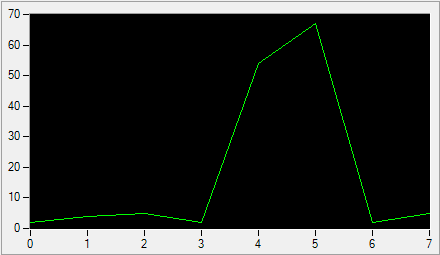• Value of DisplayMode is Samples.
• X axis label format is DateTime, â€œG5â€.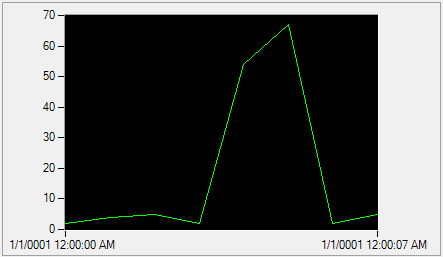• Value of DisplayMode is Time.
• X axis label format is DateTime, â€œG5â€.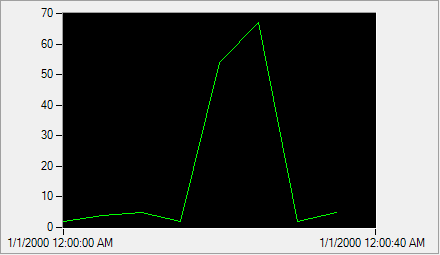• Value of DisplayMode is Time.
• X axis label format is Numeric, â€œG5â€.

The value of 6.3082E+10 in the image is the Numeric format, which is equivalent to 01/01/2000 12:00:00 AM in DateTime format.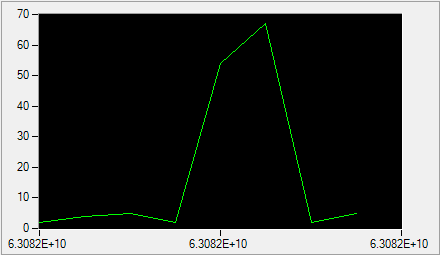• Value of DisplayMode is Time.
• X axis label format is DateTime, â€œG5â€.
• The timestamp specified by WaveformPlot.DefaultTiming.StartTime is Feb/05/2000 12:00:00 AM.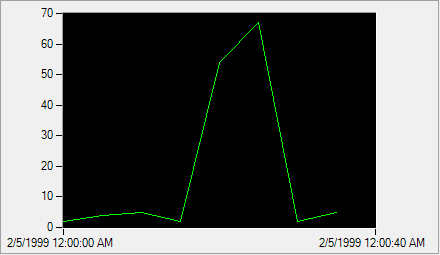• Value of DisplayMode is Time.
• X axis label format is Numeric, â€œG5â€.
• The timestamp specified by WaveformPlot.DefaultTiming.StartTime is Feb/05/2000 12:00:00 AM.